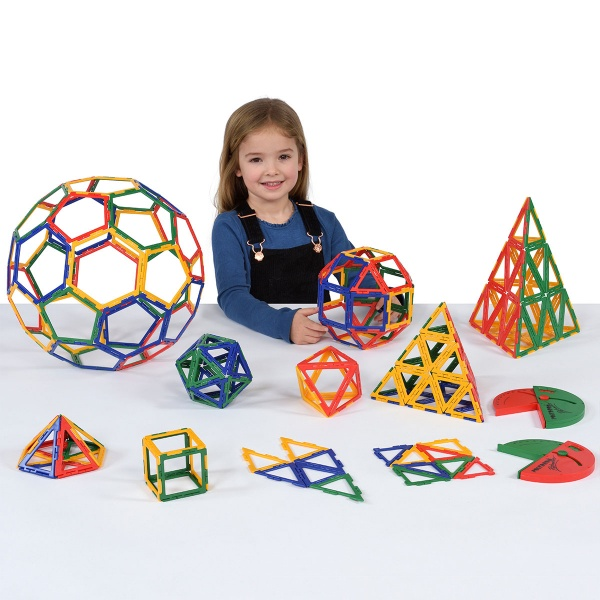# Polydron Frameworks - great for exploring shape and more...

Wednesday, 30 September 2015  |  Paul HarwoodOne of my favourite resources over the last few years has been the Polydron Frameworks Geometry Set.

When I took my first HOD role one of the first things I did was buy a set for every classroom, and more recently in my current school I was able to purchase a couple of sets to assist with our below level 4 pupils shape work. I have used it recently to explore nets and properties of 3D shapes, but actually it has been a resource I have used in lots of places in the past. Below is a list of my top uses; I have tried to spread them over all 5 strands of Maths but obviously shape gets a little bias.

Number

• Building bar models - the squares can be connected together as a physical resource to build bar models for use with fractions, decimals, percentages, ratio etc...
• Number sense - A small equilateral triangle is worth 1, the right angled triangles are worth 2, isosceles 3, large equilateral triangles 4 etc... build a shape worth x. How many different combinations of triangles equal a hexagon? How many right-triangles is a pentagon worth etc...

Shape

• Nets - Kind of obvious, but allows exploration of nets.
• Isometric drawing - Can create some interesting shapes for the more able to try and draw on isometric paper.
• Plans and Elevations - Allows real manipulation of built shapes to view their plan and elevations.
• 3D Pythag and trig - allows a real interior and exterior 3D view to calculating lengths through shapes using Pythagoras and trigonometry.
• Angle measure - the protractor in the pack allows angle measure in the flat polygons or between faces of the built 3D shapes.

Data Handling and Probability

• Probability - Put a load in a bag, what is the probability of removing a triangle? A blue shape? A blue triangle?
• Combinations and Permutations - If we have a red, blue, yellow and green triangle how many different permutations of colours can we create?

Algebra

• The area of a right triangle is a. The area of a square is b. How many ways of writing the area of two triangles and two squares are there? Other compound shapes?
• Build a 4 x 4 grid of different colour squares. If each colour is worth a different amount, what totals can we make. If this row needs to have this total, what values could they be. How many row and column values are needed to fix the value of all 4 colours.
• Sequences - Build different patterns of shapes, what is the nth term of different perimeters? Number of lines (treat a line where two shapes connect as a single line) etc.

There are lots of others which just don't come to mind right now. Please feel free to add in the comments if you can think of/have used others.

Peter Mattock, Educating Mr Mattock

 Share TweetTo contact us, call +44 (0) 1285 863980 or send us an email